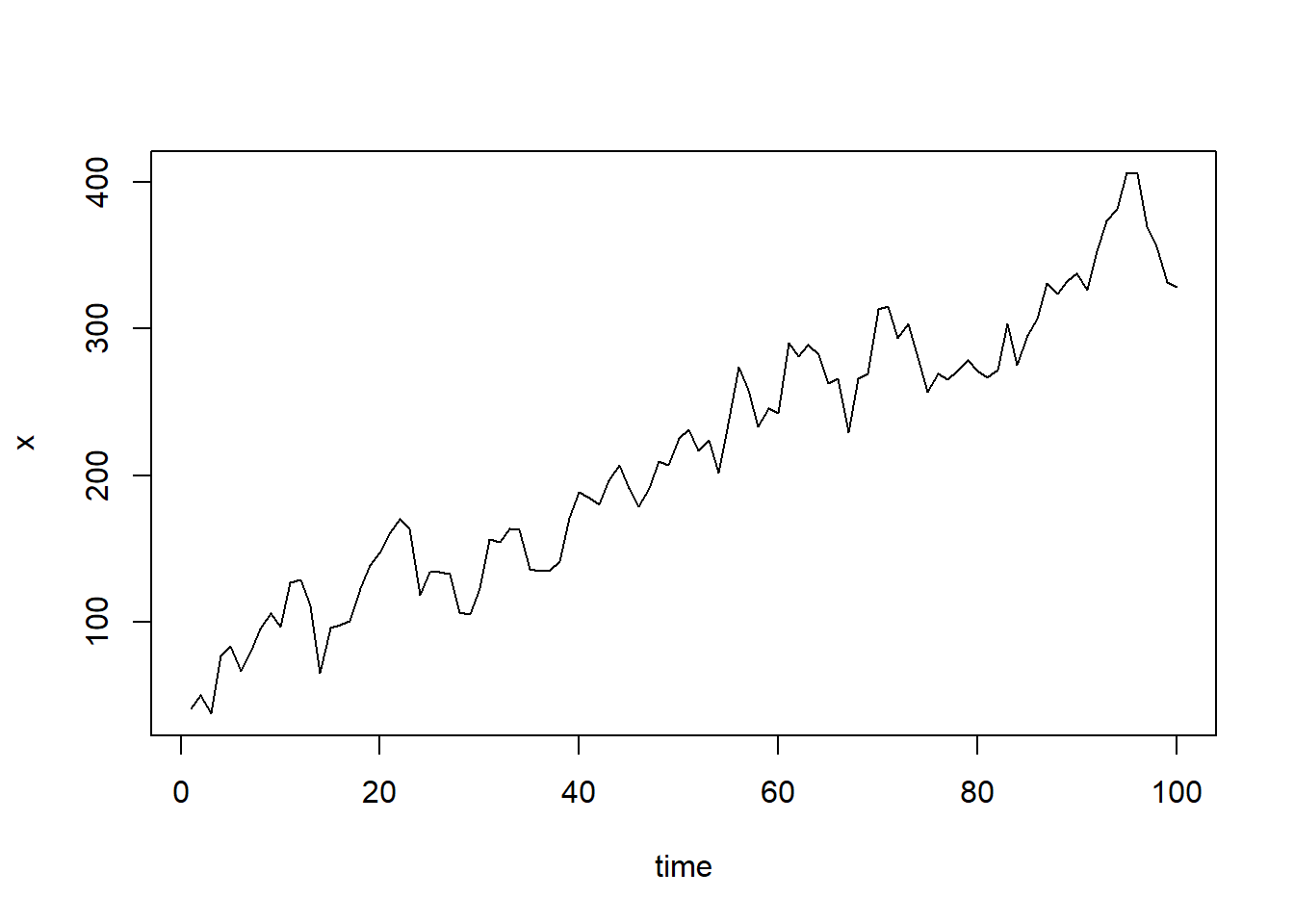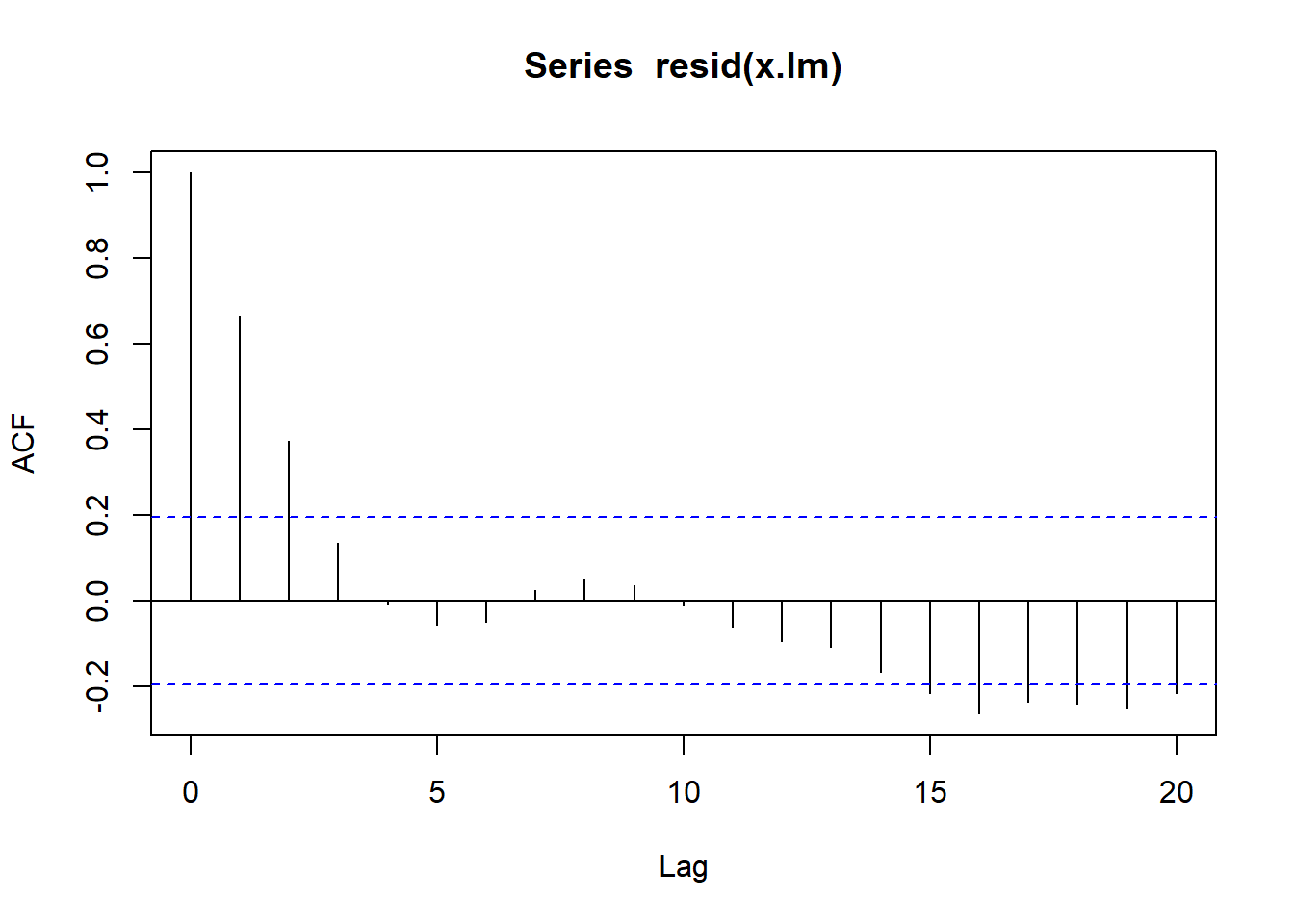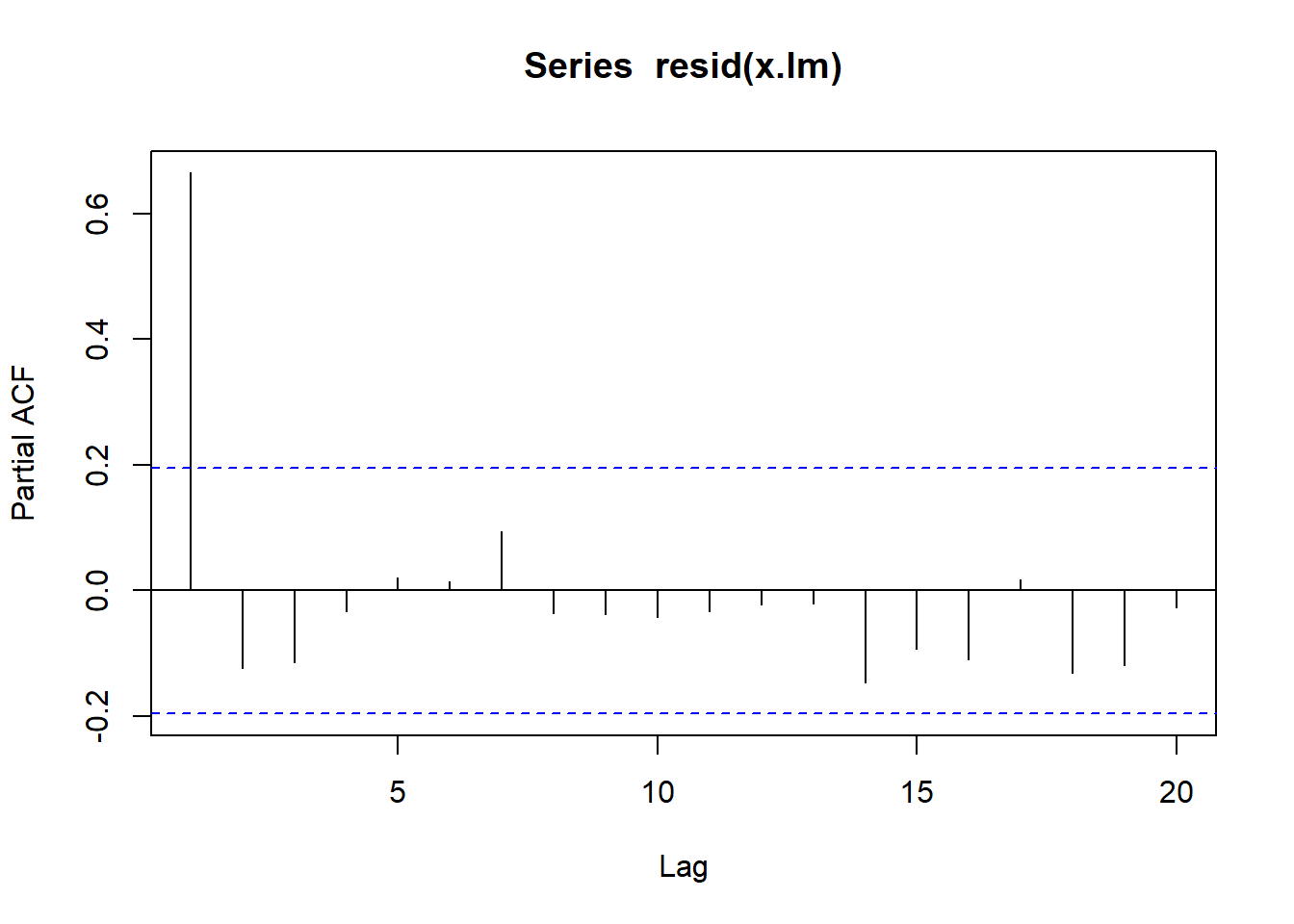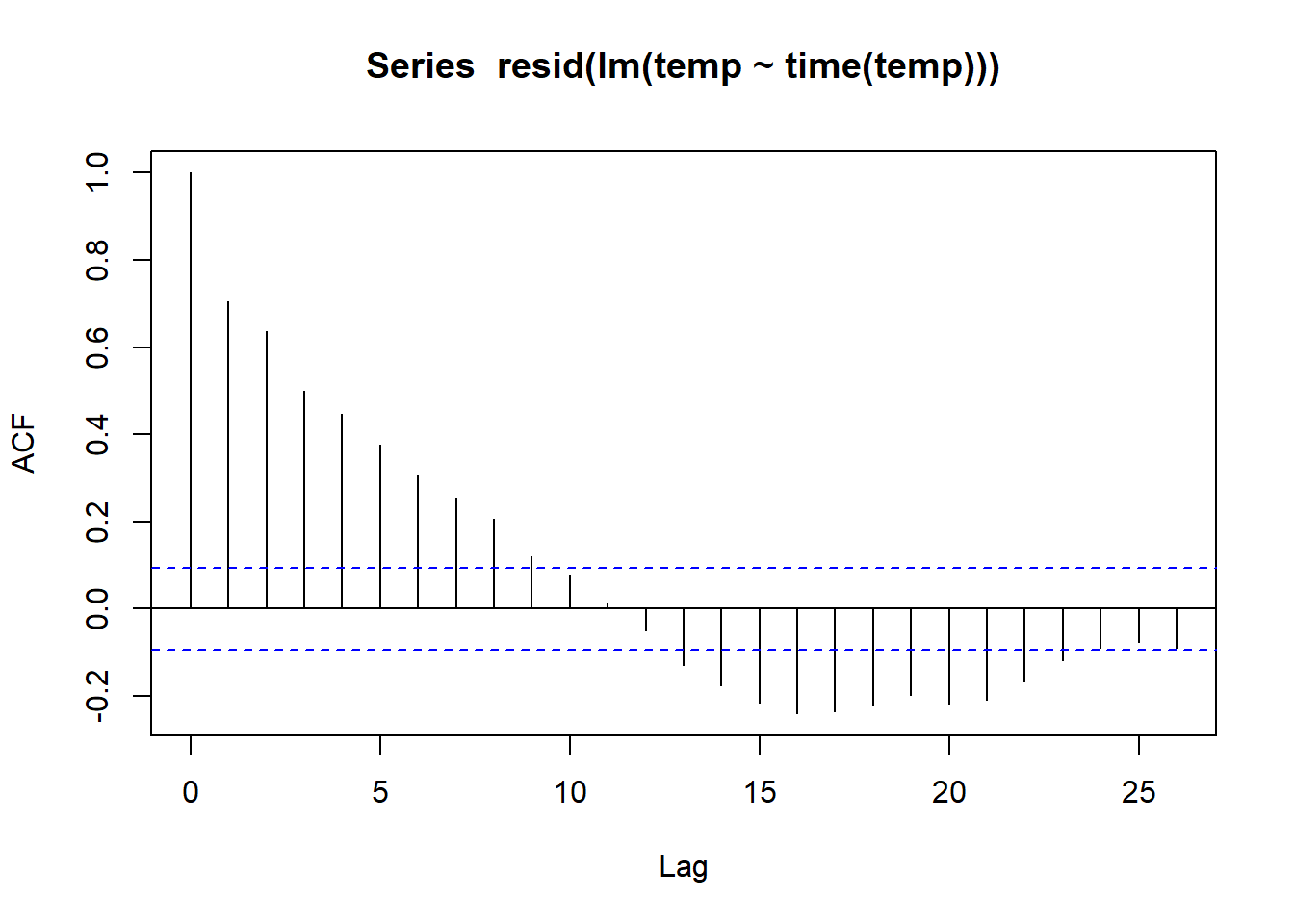load("workspace.RData")

Trends in time series can be classified as stochastic or deterministic. When we have some plausible physical explanation for a trend, we’ll usually model it in some deterministic manner. eg. the reason can be increasing population or seasonal frequency. For short term trends Linear Models are used.

## Simulation of Regression Model

It is common for error to be autocorrelated. So first we create autocorrelated errors then straight line trend is created

set.seed(1)
z <- w <- rnorm(100,sd=20)
for (t in 2:100) z[t] <- 0.8 * z[t-1]+w[t]
Time <- 1:100
x <- 50+3*Time + z
plot(x,xlab="time",type="l")Linear models are usually fitted by minimising the sum of squared errors, which can be achieved in R using the funciton lm

x.lm <- lm(x~Time)
coef(x.lm)
## (Intercept)        Time
##   58.551218    3.063275

Here the intercept is close to 50, as considered by us. Lets plot the correlograms

acf(resid(x.lm))PACF

pacf(resid(x.lm))Only the lag 1 partial autocorrelation. Which suggests that the residual series follows an AR(1) process, which was as expected.

Lets fit it to our global temperature series:

temp <- window(Global.ts,start=1970)
temp.lm <- lm(temp~time(temp))
coef(temp.lm)
## (Intercept)  time(temp)
##  -34.920409    0.017654

We can find the confidence intervals of these parameters

confint(temp.lm)
##                    2.5 %       97.5 %
## (Intercept) -37.21001248 -32.63080554
## time(temp)    0.01650228   0.01880572

The confidence interval does not contain zero, which would provide statistical evidence of an increasing trend in global temperatures. To see if it is not autocorrelated lets draw ACF of residuals

acf(resid(lm(temp~time(temp))))There is a positive autocorrelation at shorter legs, so it may be due to stochastic trends also.

## Generalized Least Square

It is common and expected that in time series the residual series be autocorrelated.

GLS can be used to provide better estimates of the residual of regression parameters.

The procedure is essentially based on maximizing the liklihood given the autocorrelation in the data.

lets fit GLS to the previous series

library(nlme)

We need the value of lag 1 autocorrelation. For historical series, the lag 1 autocorrelation would need to be estimated from the correlogram of the residuals of a fitted linear model. How: 1. Fit a linear model using OLS ( Ordinary Least Squares) 2. Read off lag 1 correlation from the correlogram plot of the residuals of the fitted model.

In this case we know it it 0.8 so we can fit now

x.gls <- gls(x~Time,cor=corAR1(0.8))
coef(x.gls)
## (Intercept)        Time
##   58.233018    3.042245

Lets see the confidence interval

confint(x.gls)
##                 2.5 %    97.5 %
## (Intercept) 34.861295 81.604742
## Time         2.645461  3.439029

Zero is not contained in the intervals, so the estimates are statistically significant and trend is significant.

save.image("workspace.Rdata")